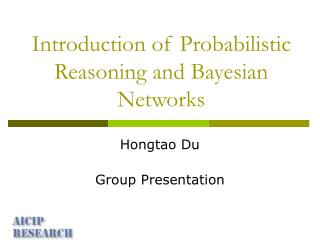DownloadDownload PresentationIntroduction of Probabilistic Reasoning and Bayesian Networks

# Introduction of Probabilistic Reasoning and Bayesian Networks

Download Presentation## Introduction of Probabilistic Reasoning and Bayesian Networks

- - - - - - - - - - - - - - - - - - - - - - - - - - - E N D - - - - - - - - - - - - - - - - - - - - - - - - - - -
##### Presentation Transcript

1. Introduction of Probabilistic Reasoning and Bayesian Networks Hongtao Du Group Presentation

2. Outline • Uncertain Reasoning • Probabilistic Reasoning • Bayesian Network (BN) • Dynamic Bayesian Network (DBN)

3. Reasoning • The activity of guessing the state of the domain from prior knowledge and observations. • Causal reasoning • Diagnostic reasoning • Combinations of these two

4. Uncertain Reasoning (Guessing) • Some aspects of the domain are often unobservable and must be estimated indirectly through other observations. • The relationships among domain events are often uncertain, particularly the relationship between the observables and non-observables.

5. The observations themselves may be unreliable. • Even though observable, very often we do not have sufficient resource to observe all relevant events. • Even though events relations are certain, very often it is impractical to analyze all of them

6. Probabilistic Reasoning • Methodology founded on the Bayesian probability theory. • Events and objects in the real world are represented by random variables. • Probabilistic models: • Bayesian reasoning • Evidence theory • Robust statistics • Recursive operators

7. Graphical Model • A tool that visually illustrate conditional independence among variables in a given problem. • Consisting of nodes (Random variables or States) and edges (Connecting two nodes, directed or undirected). • The lack of edge represents conditional independence between variables.

8. Z X Y A U B V Bayesian Network (BN) • Probabilistic network, belief network, causal network. • A specific type of graphical model that is represented as a Directed Acyclic Graph.

9. BN consists of • variables (nodes) V={1, 2, …, k} • A set of dependencies (edges) D • A set of probability distribution functions (pdf) of each variable P • Assumptions • P(X)=1 if and only if X is certain • If X and Y are mutually exclusive, then P(X v Y) = P(X) + P(Y) • Joint probability P(X, Y)= P(X|Y) P(Y)

10. X represents hypothesis • Y represents evidence • P(Y|X) is likelihood • P(X|Y) is the posterior probability • If X, Y are conditionally independent P(X|Z, Y) = P(X|Z)

11. Given some certain evidence, BN operates by propagating beliefs throughout the network. P(Z, Y, U, V) = P(Z) * P(Y|Z) * P(U|Y) * P(V|U) where is the parents of node • Explaining away • If a node is observed, its parents become dependent. • Two causes (parents) compete to explain the observed data (child).

12. Tasks in Bayesian Network • Inference • Learning

13. Inference • Inference is the task of computing the probability of each state of a node in a BN when other variables are known. • Method: dividing set of BN nodes into non-overlapping subsets of conditional independent nodes.

14. Example Given Y is the observed variable. Goal: find the conditional pdf over Case 1:

15. Case 2:

16. Learning • Goal: completing the missing beliefs in the network. • Adjusting the parameters of the Bayesian network so that the pdfs defined by the network sufficiently describes statistical behavior of the observed data.

17. M: a BN model : Parameter of probability of distribution : Observed data • Goal: Estimating to maximize the posterior probability

18. Assume is highly peaked around maximum likelihood estimates

19. Dynamic Bayesian Network (DBN) • Bayesian network with time-series to represent temporal dependencies. • Dynamically changing or evolving over time. • Directed graphical model of stochastic processes. • Especially aiming at time series modeling. • Satisfying the Markovian condition: The state of a system at time t depends only on its immediate past state at time t-1.

20. Representation • Time slice t1 t2 tk • The transition matrix that represent these time dependencies is called Conditional Probability Table (CPT).

21. Description • T: time boundary we are investigating : observable variables : hidden-state variables : state transition pdfs, specifying time dependencies between states. : observation pdfs, specifying dependencies of observation nodes regarding to other nodes at time slice t. : initial state distribution.

22. Tasks in DBN • Inference • Decoding • Learning • Pruning

23. Inference • Estimating the pdf of unknown states through given observations and initial probability distributions. • Goal: finding : a finite set of T consecutive observations : the set of corresponding hidden variables

24. Decoding • Finding the best-fitting probability values for the hidden states that have generated the known observations. • Goal: determine the sequence of hidden states with highest probabilities.

25. Learning • Given a number of observations, estimating parameters of DBN that best fit the observed data. • Goal: Maximizing the joint probability distribution. : the model parameter vector

26. Pruning • An important but difficult task in DBN. • Distinguishing which nodes are important for inference, and removing the unimportant nodes. • Actions: • Deleting states from a particular node • Removing the connection between nodes • Removing a node from the network

27. Time slice t • : designated world nodes, a subset of the nodes, representing the part we want to inspect. • , If state of is known, , then are no longer relevant to the overall goal of the inference. Thus, (1) delete all nodes (2) incorporate knowledge that

28. Future work • Probabilistic reasoning in multiagent systems. • Different DBNs and applications. • Discussion of DBN problems.